# Dynamic Day CalculationHi,

We're struggling with a conceptually easy topic which is surprisingly difficult to model build in Anaplan.

For a dynamic ordering calendar, so the days when you can order can be adjusted and are not fixed, we're looking to define the amount of days in between two ordering days.

Technically I'm stuck at the point where you have to get the pull back the amount of days to the 'ordering day'.

1. Days you can order = IF Days you can order Boolean = TRUE THEN 1 ELSE 0

2. Calculation Line =  IF Days you can order = 0 THEN 1 ELSE 0

3. Days until next ordering day = CUMULATE(Calculation Line, Days you can order Boolean)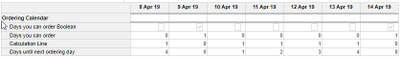Now an extra line item should be built which pulls forward the 4 on 13 Apr 19 and puts it on 9 Apr 19.

Would be of tremendous value if someone could help to configure the line item formula.

Thank you!

Hendrik

Tagged:

•Try this,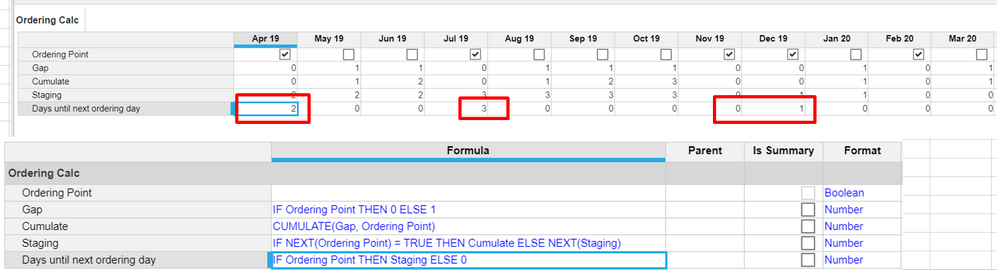I hope this gives the result you are expecting.

~Vignesh M

•A slightly different solution: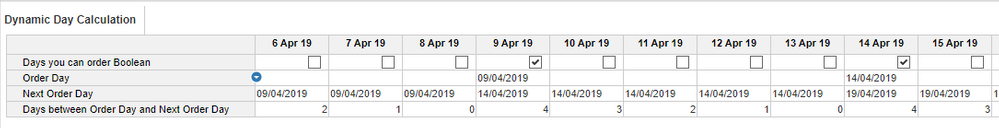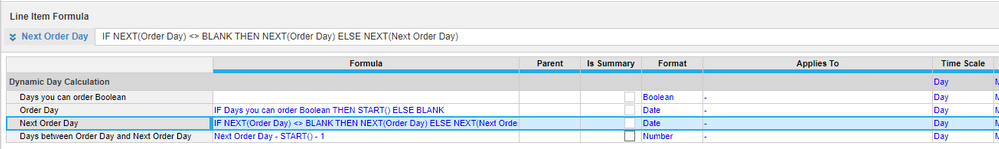•I know I am late to the game here, but here is another way of doing it: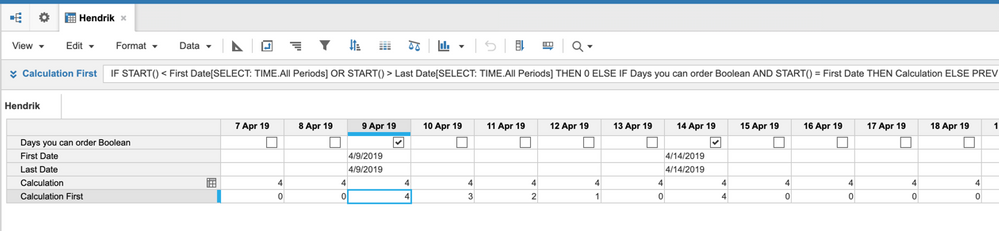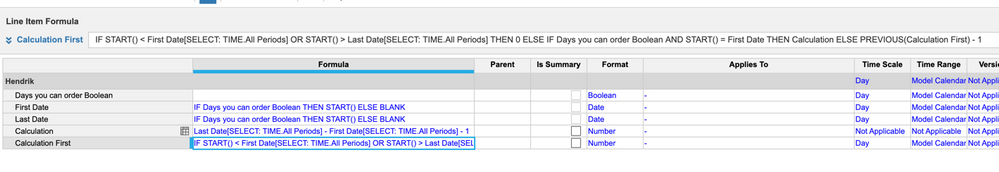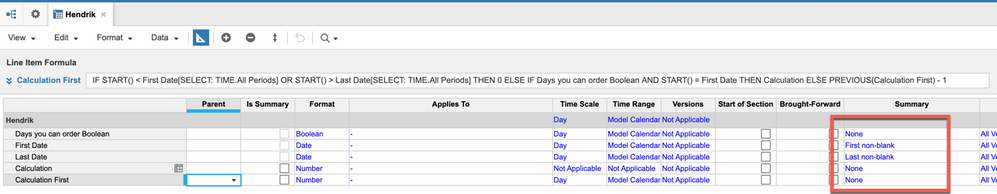Formulas for the line items:

First Date: IF Days you can order Boolean THEN START() ELSE BLANK

Last Date: IF Days you can order Boolean THEN START() ELSE BLANK

Calculation: Last Date[SELECT: TIME.All Periods] - First Date[SELECT: TIME.All Periods] - 1

Calculation First: IF START() < First Date[SELECT: TIME.All Periods] OR START() > Last Date[SELECT: TIME.All Periods] THEN 0 ELSE IF Days you can order Boolean AND START() = First Date THEN Calculation ELSE PREVIOUS(Calculation First) - 1

Rob

•Can i assume, you are trying to calculate, number of days between the line item Days you can order - Which is set as True.

Eg: in your example Days you can order is set as true on 9 and 14th April 2019. -> 4 days is between 9 and 14th April.....?

Is that the result that you are expecting on 9th April....?

•Hi,

Yes, that should be the end result!
•Hi,

Thank you both for the quick response!

Appreciate the help, works like a charm.

Kind regards,

Hendrik

•Hi Rob,

Thanks for taking a jab at this as well!

Seems perfect.

Have a good day!

Hendrik

•Hi Rob,

Just realized this approach wouldn't work I think as you assume there are only two dates to take into account.

However this can vary and will mainly be around two times every week, but might be 3 or even 0.

As you're using the SELECT: Time.All Periods it wouldn't allow for multiple ordering dates, unless I got that wrong.

No need to adjust but just wanted to share my thoughts!

Thanks again for helping.

Hendrik

•You are correct, I assumed it would only be two dates which is where the SELECT All Time Periods comes into play.  If you have multiple, you are correct in that you would either have to alter this solution or go with a previous solution provided.

Rob